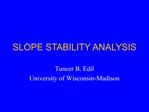# SLOPE STABILITY ANALYSIS - PowerPoint PPT PresentationDownload PresentationSLOPE STABILITY ANALYSIS

SLOPE STABILITY ANALYSISDownload Presentation## SLOPE STABILITY ANALYSIS

- - - - - - - - - - - - - - - - - - - - - - - - - - - E N D - - - - - - - - - - - - - - - - - - - - - - - - - - -
##### Presentation Transcript

1. SLOPE STABILITY ANALYSIS Tuncer B. Edil University of Wisconsin-Madison

2. LECTURE OUTLINE Common Features of Slope Stability Analysis Methods Water Forces on Soil Infinite Slope Analysis Finite Slopes: Plane, Circular and Noncircular Failure Surfaces

3. COMMON FEATURES OF SLOPE STABILITY ANALYSIS METHODS Safety Factor: F = S/Sm where S = shear strength and Sm = mobilized shear resistance. F = 1: failure, F > 1: safety Shape and location of failure is not known a priori but assumed (trial and error to find minimum F) Static equilibrium (equilibrium of forces and moments on a sliding mass) Two-dimensional analysis

4. INFINITE SLOPE ANALYSIS Translational failures along a single plane failure surface parallel to slope surface The ratio of depth to failure surface to length of failure zone is relatively small (<10%) Applies to surface raveling in granular materials or slab slides in cohesive materials Equilibrium of forces on a slice of the sliding mass along the failure surface is considered

5. INFINITE SLOPE